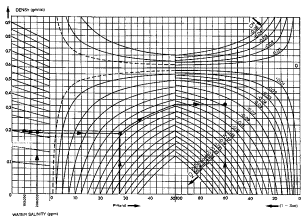Iterative and Error Minimization Solutions
One outcome of the hydrocarbon density calculation is the possibility that all prior work is wrong because the wrong fluid density has been used in the shale volume and porosity equations, resulting in an incorrect water saturation as well.Iterative Methods
Some programs, notably the service company well site computer programs and their in-house counterparts perform a series of iterative solutions to overcome this. The procedure would be as follows:

1. Apply environmental corrections to raw log data.

2. Calculate shale volume from any appropriate method.

3. Calculate porosity from any appropriate method.

5. Calculate hydrocarbon and apparent fluid density.

6. Calculate correction to density and neutron logs, if fluid density is less than 0.8 gm/cc 800 kg/m3Gas corrections for density (above) and neutron logs (below) for iterative models.7. Add these corrections to the previous density and neutron log data.

8. Re-compute shale volume if selected method used density or neutron log data, using the new density and neutron values.

9. Re-compute porosity if selected method used density or neutron log data, using the new density, neutron and new shale volume.

10. Re-compute saturation using the new porosity and shale volume.

11. Compare new porosity with previous value.

12. If there is little change, then go to step 13, otherwise repeat steps 5 through 12.

13. Calculate other results desired with the final shale, porosity and saturation values.

This process is indicated in the flow diagram at right,

Since other parameters may be in error, particularly shale and matrix assumptions, the iteration may not converge, so a trap must be put in the program to exit after some reasonable number of iterations defined by the user. Even if it does converge, the result may still suffer from poor data or poor assumptions.

Due to gas effect on sonic data, iteration could improve results from this method provided some relationship could be quantified between Sxo and the fluid travel time. This relationship is not well known and is not likely to be helpful at the present time.Error Minimization Methods
Error minimization solutions are also iterative methods. Computer products such as Schlumberger's GLOBAL and Gearhart's ULTRA are programs of this type.

The steps taken are as follows:

1. Apply environmental corrections to raw log data.

2. Calculate shale volume from any appropriate method.

3. Calculate porosity from any appropriate method.

5. Calculate lithology and mineral fractions (see Chapter Nine)

6. Calculate theoretical log response based on above answers from appropriate log response equations.

7. Compare calculated response with actual logs and calculate error statistics.

8. Sum these errors and compare to previous iterations.

9. If error is minimum go to Step 11

10. If error is not minimum, adjust analysis parameters and analysis model and repeat Steps 2 through 10.

11. Calculate other results desired with the final shale, porosity and saturation values.

If this method is used in an interactive program, the analyst can be involved in the decision process of Step 10. If the computer program contains the decision making logic, it works best when the analyst has described the best model, and may fail to give reasonable results when an inappropriate model has been presented.

Page Views ---- Since 01 Jan 2015